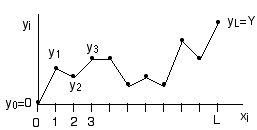Home

PHY 418: Statistical Mechanics I
Prof. S. Teitel stte@pas.rochester.edu ---- Spring 2018

## Problem Set 4

Due Monday, March 19, in lecture

• Problem 1 [20 points]

In lecture we discussed the canonical ensemble, in which the temperature T, volume V, and number of particle N of a system are fixed, while the energy E is allowed to fluctuate. Suppose now that you wish to describe a system in which the temperature T, number of particles N, and pressure p are fixed, while the volume V is allowed to fluctuate. This would describe a system in contact with a thermal and mechanical reservior, in which the wall separating the system and the reservior is heat conducting and moveable. We can call this case the constant pressure ensemble.

a) [5 pts] Define the appropriate partition function Z(T, p, N) of the system in this new constant pressure ensemble.

b) [5 pts] If you defined Z properly in part (a), then the Gibbs free energy should be given by

G(T, p, N) = -kBT ln Z(T, p, N)

To demonstrate this, show that using G defined from Z as above, the average volume of the system is correctly given by,

<V> = (∂G/∂p)T,N

c) [5 pts] Derive a relation, in this constant pressure ensemble, between the isothermal compressibility κT and fluctuations in the volume V of the system. Show from this relation that the relative fluctuation in V vanishes in the thermodynamic limit.

d) [5 pts] Consider an ideal gas of indistinguishable, non-relativistic, non-interacting, point particles of mass m. Explicitly compute the partition function Z(T,p,N) of this gas. Use Z to compute G(T,p,N), and then from G compute the specific heat at constant pressure, Cp. Show that you get the correct answer for the ideal gas.

• Problem 2 [30 points]

This problem is meant to get you to think about the relationship between different ensembles and their corresponding free energies, and how one can often change ensembles to remove a constraint and make a calculation simpler.

Consider the following model for an elastic string in a plane. The height of the string at position x is the function y(x). To make the calculation doable, imagine discretizing the horizontal axis into L discrete units, xi=i, i=0, 1, 2, ..., L. The height at position xi is then yi.The energy to stretch the string is then determined by the height differences according to the following Hamiltonian:

 H[yi] = (1/2) α L∑i=1 (yi - yi-1)2

a) [15 pts] The string is constrained so that its endpoints are fixed at:

y0=0,       yL=Y

so that the string has an average slope Y/L.

Compute the partition function

 Z(Y) = ( L-1 ∏i=1 +∞∫-∞ dyi) e-H[yi]/kBT

Compute the corresponding free energy,

F(Y) = - kBT ln Z(Y)

There are two tricks you need to sum the partition function. One is to figure out how to deal with the fact that yi interacts with both yi+1 and yi-1. The second is to figure out how to deal with the constraint of the fixed enpoints. If you get desparate, here is a hint.

b) [15 pts] Now consider the partition function in a new ensemble

 Z'(η) = +∞∫-∞ dY Z(Y) e-η Y/kBT

Z'(η) is the Laplace transform of Z(Y).

In this ensemble, the average slope of the string, (yL-y0)/L is no longer fixed, but fluctuates. Its average value, however, is determined by the parameter η.

Compute Z'(η) directly by plugging the definition of Z(Y) from (a) into the above integral and then summing over the degrees of freedom (i.e. do NOT use your result for Z(Y) from part (a) and take the Laplace transform of it). You should find that it is now much easier to sum over the degrees of freedom because the constraint yL=L is no longer present.

Having found Z'(η) compute the corresponding free energy

F'(η) = -kBT ln Z'(η)

Show that the average height difference of the endpoints, Y=yL-y0, is given by

 = ∂F'(η)/∂η

Now take the Legendre transform of F'(η) to get,

F(Y) = F'(η) - ηY

Show that F(Y) above agrees with what you found in part (a), provided you take the thermodynamic limit of L → ∞. That is, show that the free energies per unit length, F(Y)/L, are equal as L → ∞.

You should find that the calculation in (b) is much easier and more straight forward to do than the calculation in (a). This shows that by going to the new ensemble, in which the original constraint of fixed Y was lifted by introducing its conjugate variable η, the calculation of F(Y) becomes much easier.